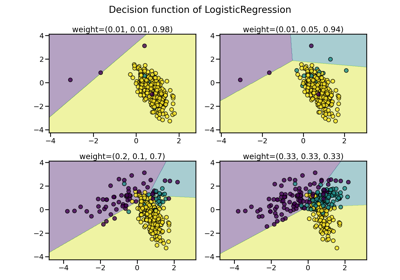# SMOTEN#

class imblearn.over_sampling.SMOTEN(*, sampling_strategy='auto', random_state=None, k_neighbors=5, n_jobs=None)[source]#

Synthetic Minority Over-sampling Technique for Nominal.

This method is referred as SMOTEN in . It expects that the data to resample are only made of categorical features.

Read more in the User Guide.

New in version 0.8.

Parameters
sampling_strategyfloat, str, dict or callable, default=’auto’

Sampling information to resample the data set.

• When float, it corresponds to the desired ratio of the number of samples in the minority class over the number of samples in the majority class after resampling. Therefore, the ratio is expressed as $$\alpha_{os} = N_{rm} / N_{M}$$ where $$N_{rm}$$ is the number of samples in the minority class after resampling and $$N_{M}$$ is the number of samples in the majority class.

Warning

float is only available for binary classification. An error is raised for multi-class classification.

• When str, specify the class targeted by the resampling. The number of samples in the different classes will be equalized. Possible choices are:

'minority': resample only the minority class;

'not minority': resample all classes but the minority class;

'not majority': resample all classes but the majority class;

'all': resample all classes;

'auto': equivalent to 'not majority'.

• When dict, the keys correspond to the targeted classes. The values correspond to the desired number of samples for each targeted class.

• When callable, function taking y and returns a dict. The keys correspond to the targeted classes. The values correspond to the desired number of samples for each class.

random_stateint, RandomState instance, default=None

Control the randomization of the algorithm.

• If int, random_state is the seed used by the random number generator;

• If RandomState instance, random_state is the random number generator;

• If None, the random number generator is the RandomState instance used by np.random.

k_neighborsint or object, default=5

The nearest neighbors used to define the neighborhood of samples to use to generate the synthetic samples. You can pass:

• an int corresponding to the number of neighbors to use. A ~sklearn.neighbors.NearestNeighbors instance will be fitted in this case.

• an instance of a compatible nearest neighbors algorithm that should implement both methods kneighbors and kneighbors_graph. For instance, it could correspond to a NearestNeighbors but could be extended to any compatible class.

n_jobsint, default=None

Number of CPU cores used during the cross-validation loop. None means 1 unless in a joblib.parallel_backend context. -1 means using all processors. See Glossary for more details.

Deprecated since version 0.10: n_jobs has been deprecated in 0.10 and will be removed in 0.12. It was previously used to set n_jobs of nearest neighbors algorithm. From now on, you can pass an estimator where n_jobs is already set instead.

Attributes
sampling_strategy_dict

Dictionary containing the information to sample the dataset. The keys corresponds to the class labels from which to sample and the values are the number of samples to sample.

nn_k_estimator object

Validated k-nearest neighbours created from the k_neighbors parameter.

n_features_in_int

Number of features in the input dataset.

New in version 0.9.

feature_names_in_ndarray of shape (n_features_in_,)

Names of features seen during fit. Defined only when X has feature names that are all strings.

New in version 0.10.

SMOTE

Over-sample using SMOTE.

SMOTENC

Over-sample using SMOTE for continuous and categorical features.

BorderlineSMOTE

Over-sample using the borderline-SMOTE variant.

SVMSMOTE

Over-sample using the SVM-SMOTE variant.

ADASYN

KMeansSMOTE

Over-sample applying a clustering before to oversample using SMOTE.

Notes

See the original papers:  for more details.

Supports multi-class resampling. A one-vs.-rest scheme is used as originally proposed in .

References

1(1,2,3)

N. V. Chawla, K. W. Bowyer, L. O.Hall, W. P. Kegelmeyer, “SMOTE: synthetic minority over-sampling technique,” Journal of artificial intelligence research, 321-357, 2002.

Examples

>>> import numpy as np
>>> X = np.array(["A"] * 10 + ["B"] * 20 + ["C"] * 30, dtype=object).reshape(-1, 1)
>>> y = np.array( * 20 +  * 40, dtype=np.int32)
>>> from collections import Counter
>>> print(f"Original class counts: {Counter(y)}")
Original class counts: Counter({1: 40, 0: 20})
>>> from imblearn.over_sampling import SMOTEN
>>> sampler = SMOTEN(random_state=0)
>>> X_res, y_res = sampler.fit_resample(X, y)
>>> print(f"Class counts after resampling {Counter(y_res)}")
Class counts after resampling Counter({0: 40, 1: 40})


Methods

 fit(X, y) Check inputs and statistics of the sampler. fit_resample(X, y) Resample the dataset. get_feature_names_out([input_features]) Get output feature names for transformation. get_params([deep]) Get parameters for this estimator. set_params(**params) Set the parameters of this estimator.
fit(X, y)[source]#

Check inputs and statistics of the sampler.

You should use fit_resample in all cases.

Parameters
X{array-like, dataframe, sparse matrix} of shape (n_samples, n_features)

Data array.

yarray-like of shape (n_samples,)

Target array.

Returns
selfobject

Return the instance itself.

fit_resample(X, y)[source]#

Resample the dataset.

Parameters
X{array-like, dataframe, sparse matrix} of shape (n_samples, n_features)

Matrix containing the data which have to be sampled.

yarray-like of shape (n_samples,)

Corresponding label for each sample in X.

Returns
X_resampled{array-like, dataframe, sparse matrix} of shape (n_samples_new, n_features)

The array containing the resampled data.

y_resampledarray-like of shape (n_samples_new,)

The corresponding label of X_resampled.

get_feature_names_out(input_features=None)[source]#

Get output feature names for transformation.

Parameters
input_featuresarray-like of str or None, default=None

Input features.

• If input_features is None, then feature_names_in_ is used as feature names in. If feature_names_in_ is not defined, then the following input feature names are generated: ["x0", "x1", ..., "x(n_features_in_ - 1)"].

• If input_features is an array-like, then input_features must match feature_names_in_ if feature_names_in_ is defined.

Returns
feature_names_outndarray of str objects

Same as input features.

get_params(deep=True)[source]#

Get parameters for this estimator.

Parameters
deepbool, default=True

If True, will return the parameters for this estimator and contained subobjects that are estimators.

Returns
paramsdict

Parameter names mapped to their values.

set_params(**params)[source]#

Set the parameters of this estimator.

The method works on simple estimators as well as on nested objects (such as Pipeline). The latter have parameters of the form <component>__<parameter> so that it’s possible to update each component of a nested object.

Parameters
**paramsdict

Estimator parameters.

Returns
selfestimator instance

Estimator instance.

## Examples using imblearn.over_sampling.SMOTEN#Compare over-sampling samplers

Compare over-sampling samplers